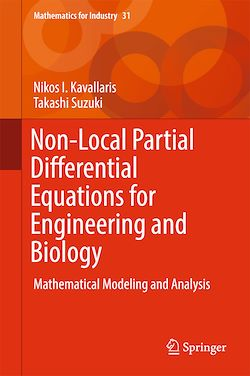# Non-Local Partial Differential Equations for Engineering and Biology

-English
300 Pages

Description

This book presents new developments  in non-local mathematical modeling and mathematical analysis on the behavior of solutions with novel technical tools. Theoretical backgrounds in mechanics, thermo-dynamics, game theory, and theoretical biology are examined in details. It starts off with a review and summary of the basic ideas of mathematical modeling frequently used in the sciences and engineering. The authors then employ a number of models in bio-science and material science to demonstrate applications, and provide recent advanced studies, both on deterministic non-local partial differential equations and on some of their stochastic counterparts used in engineering. Mathematical models applied in engineering, chemistry, and biology are subject to conservation laws. For instance, decrease or increase in thermodynamic quantities and non-local partial differential equations, associated with the conserved physical quantities as parameters. These present novel mathematical objects are engaged with rich mathematical structures, in accordance with the interactions between species or individuals, self-organization, pattern formation, hysteresis. These models are based on various laws of physics, such as mechanics of continuum, electro-magnetic theory, and thermodynamics. This is why many areas of mathematics, calculus of variation, dynamical systems, integrable systems, blow-up analysis, and energy methods are indispensable in understanding and analyzing these phenomena.
This book aims for researchers and upper grade students in mathematics, engineering, physics, economics, and biology.

Subjects

##### Differential equation

Informations